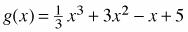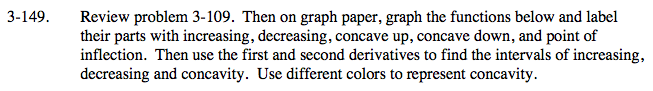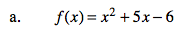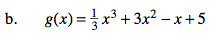### Home > CALC > Chapter 3 > Lesson 3.4.1 > Problem3-149

3-149.
1. Review problem 3-109. Then on graph paper, graph the functions below and label their parts with increasing, decreasing, concave up, concave down, and point of inflection. Then use the first and second derivatives to find the intervals of increasing, decreasing and concavity. Use different colors to represent concavity. Homework Help ✎

1. f(x) = x2 + 5x − 6

2.f '(x) = 2x + 5 Power rule.
f '(x) = 2

To find where f(x) is increasing or decreasing , set the derivative equal to zero:
0 = f '(x)
0 = 2x + 5

$-\frac{5}{2}=x$

This is the location of a local max or min.

Now determine where f(x) is increasing or decreasing:

$\text{When }x< -\frac{5}{2},\ f'(x)< 0$

$\text{When }x>-\frac{5}{2},\ f'(x)> 0$

To find where f(x) is concave up or concave down, first find the location of the point of inflection:
0 = f "(x)
0 = 2 no point of inflection
In fact: f "(x) = 2, so f "(x) > 0 always. That means that f(x) is always concave up. (Which makes sense because f(x) = x2 + 5x − 6, an upward facing parabola.)Follow the hints in part (a). But be aware that this function will have an inflection point. That means that, along with several changes in slope, there will be a change in concavity.